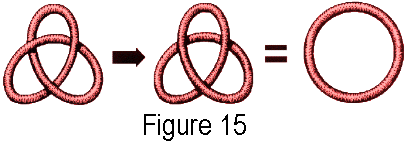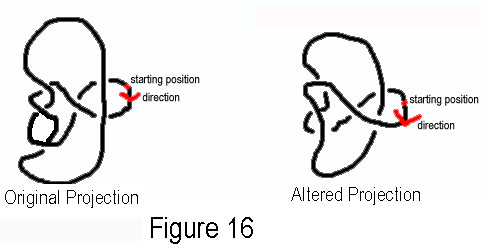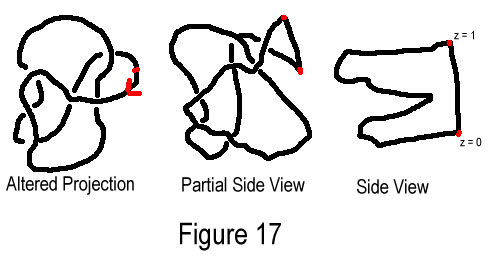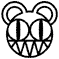# EXAMPLES OF INVARIANTS:

## THE UNKNOTTING NUMBER

Adams defines the unknotting number, another invariant, like this:
A knot K has unknotting number n if there exists a projection of the knot such that changing n crossings in the projection turns the knot into the unknot and there is no projection such that fewer changes would have turned it into the unknot.See figure 15 for an example of this.  I could not find a proof that the unknotting number is in fact an invariant.  Notice that this definition assumes that there are a finite number of uncrossings that can be applied to any knot to make it into the unknot.  We will prove this using results from Adams.First of all, we need to choose a starting point on the knot that is not at a crossing, and start traveling in a particular direction along the knot.  Arriving at our first crossing, we observe whether the strand we are on is the overstrand.  If not, we change the crossing.  We continue in this fashion unless we reach a crossing where we have already been.  In this case, we leave the crossing as it is, and continue on what must be the understrand.  When we reach the starting point, we have produced a knot which is easy to show is the unknot.  See figure 16 for a visual image of what was just described.Applying an x-y-z-coordinate system to the knot in 3-space with the z-axis coming straight out towards us, assume that the initial point is at coordinate z = 1.  Continuing to trace along the knot, at each crossing we will decrease the z-coordinate at that point, until the last point will have coordinate z = 0.  But the last point is the same as the first point, so we must put a vertical bar from the last point to the first point to complete the knot.  Now, as demonstrated more clearly in figure 17, as we examine the knot straight down the z-axis, the knot has no crossings.  That is, the knot is the unknot.  Thus, we have shown that any arbitrary knot has a finite unknotting number. 

Next Example: The linking numberkMain menu      1. History              2.Intro               3.Invariants     4.Composition    5.Conclusion